#### Completing the state transition equation

Assume that the body frame of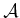aligns with the principle axes. The remaining six equations of motion can finally be given in a nice form. Using (13.99), the expression (13.98) reduces to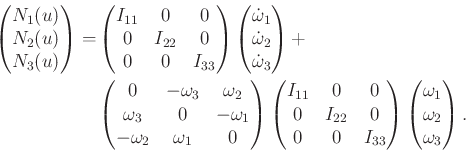(13.100)

Multiplying out (13.100) yields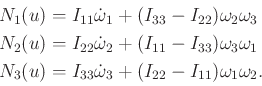(13.101)

To prepare for the state transition equation form, solving for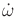yields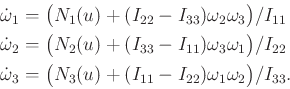(13.102)

One final complication is that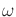needs to be related to angles that are used to express an element of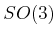. The mapping between these depends on the particular parameterization of. Suppose that quaternions of the form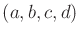are used to express rotation. Recall that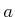can be recovered once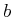,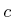, and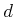are given using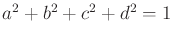. The relationship betweenand the time derivatives of the quaternion components is obtained by using (13.84) (see , p. 433):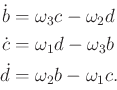(13.103)

This finally completes the specification of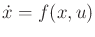, in which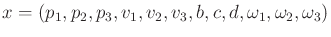(13.104)

is a twelve-dimensional phase vector. For convenience, the full specification of the state transition equation is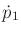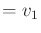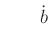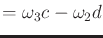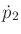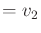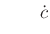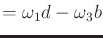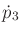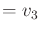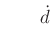(13.105)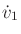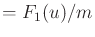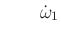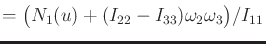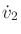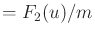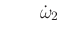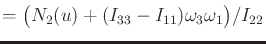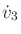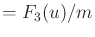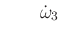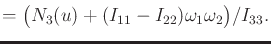The relationship between inertia matrices and ellipsoids is actually much deeper than presented here. The kinetic energy due to rotation only is elegantly expressed as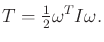(13.106)

A fascinating interpretation of rotational motion in the absence of external forces was given by Poinsot [39,681]. As the body rotates, its motion is equivalent to that of the inertia ellipsoid, given by (13.106), rolling (without sliding) down a plane with normal vector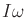in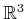.

Steven M LaValle 2020-08-14